### Home > A2C > Chapter 9 > Lesson 9.3.2 > Problem9-158

9-158.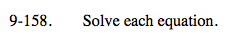$x=\frac{5}{9}$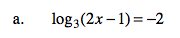Convert the equation into exponential form.
3−2 = 2x − 1

Now solve for x.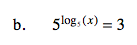Remember the rule b logb(N) = N

x = 3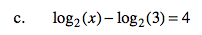Remember the rule that log(N)−log(M) = log(N/M).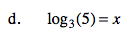3x = 5